## Monday, November 9, 2015

### Types of Quadrilaterals

There are six basic types of quadrilaterals:
###### 1.  Rectangle
Opposite sides of a rectangle are parallel and equal.  All angles are 90º.
###### 2.  Square
Opposite sides of a square are parallel and all sides are equal.  All angles are 90º.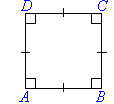###### 3.  Parallelogram
Opposite sides of a parallelogram are parallel and equal.  Opposite angles are equal.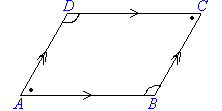###### 4.  Rhombus
All sides of a rhombus are equal and opposite sides are parallel.  Opposite angles of a rhombus are equal.  The diagonals of a rhombus bisect each other at right angles.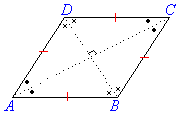###### 5.  Trapezium
trapezium has one pair of opposite sides parallel.  A regular trapezium has non-parallel sides equal and its base angles are equal, as shown in the following diagram.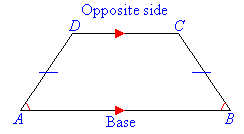###### 6.  Kite
Two pairs of adjacent sides of a kite are equal, and one pair of opposite angles are equal.   Diagonals intersect at right angles.  One diagonal is bisected by the other.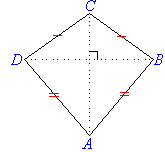###### 4.  Rhombus
All sides of a rhombus are equal and opposite sides are parallel.  Opposite angles of a rhombus are equal.  The diagonals of a rhombus bisect each other at right angles.###### 5.  Trapezium
trapezium has one pair of opposite sides parallel.  A regular trapezium has non-parallel sides equal and its base angles are equal, as shown in the following diagram.###### 6.  Kite
Two pairs of adjacent sides of a kite are equal, and one pair of opposite angles are equal.   Diagonals intersect at right angles.  One diagonal is bisected by the other.